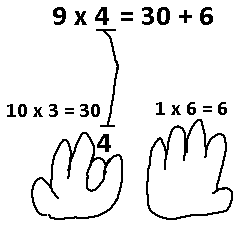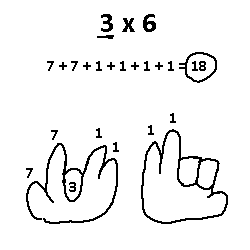# Generalizing the Hand Multiply Trick

Have you learned the multiply by 9 with your hands trick before? There is a way to find the product of 9 and any single digit number using just your hands. For those that do not know, here’s how it works. Say you want to multiply 9 by 7. You count (from left to right) to your 7th finger, and put that finger down. Every finger to the left counts as 10 and every finger to the right counts as 1. You will have 6 fingers up on the left and 3 up on the right, hence 63. Below is another example.Now there is actually no reason why we have to limit ourselves to only multiplying numbers by 9! The trick can be generalized to multiply any 2 single digit numbers together, and the reasoning has to do with how many fingers you have on your hand. As you will see, the multiply by 9 trick works because we have 10 fingers on our hands.

Just to warn you right now, using this trick is probably more trouble to use than to just memorize your times tables, but as someone who is doing recreational math I don’t care about practicality. Let’s continue.

Let’s start with a concrete example. How to multiply 3 by 6? First, take the larger number (6), and count on your fingers, left to right, to 6 plus 1. That seventh finger should be up, but every finger after that should be put down. Pretend you only have 7 fingers now. Note that this general case still makes sense to the 9 trick, since the 10th finger would’ve been up, but all fingers after that (there are none) are excluded.Now take that 3 and do exactly what we did with the 9 trick. The third finger goes down, and now you have two sets of fingers. All fingers to the right of that third finger are still counted as 1’s, but now all fingers on the left side are counted in 7’s. It all depends on the last finger on your hand that is still up, which is the 7th. From here it is just arithmetic.Here’s the general algorithm: Assume we have two single-digit numbers, n and m, where n is the larger number. First, go to the (n+1)th finger, and all fingers to the right of that one go down and are excluded. Next, go to the mth finger, and put that one down. Each finger to the left of the mth finger has a value of n + 1, and each finger to the right of the mth finger has a value of 1.

Try it out using your hands. There’s only one last thing to show.

Surely we can format the process here into a formula, right? Right. We know the values of all the fingers based on n and m, but how do we know how many fingers will have these values? Let’s start with the left side of the mth finger. Each finger has a value n + 1. Note that the number of fingers depends exclusively on m, since the mth finger decides the cutting line. In general, the left side will have (m-1) fingers. Multiplying the number of fingers (m-1) by their value (n+1) gives us (m-1)(n+1) for the first value.

The right set of fingers have only a value of 1, and are dependent on both n and m. One way to count the number of fingers on the right side is to include all fingers up to finger n + 1 (we keep an extra finger up on the right!), then remove all the fingers that aren’t on the right side, which would be m fingers. So, that would be n + 1 - m fingers on the right side, all with values of 1. Multiplying something by 1 doesn’t mean anything, so that means we’re done here.

Time to sum up all the values! The left side is (m-1)(n+1) and the right side is (n+1-m). Now we add them together and the result is…

(m-1)(n+1) + (n+1-m)

At this point, the relationship between n and m doesn’t matter. They don’t have to be single digits and one doesn’t need to be greater than the other. In fact, if we simplify this equation, we should get back the product of n and m, our original goal.

(m*n + m - n - 1) + n + 1 - m

Everything cancels out except for…. m * n. That’s basically it.